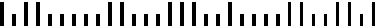AN OVERVIEW OF POSTNET

H. Roumani - Winter 1999

Documents requiring fast, automated processing, like cheques, envelopes and supermarket items, are often encoded to make key data fields machine-readable. The US Postal Service, for example, uses the POSTNET (Postal Numeric Encoding Technique) bar code to encode zip codes on letters. The zip code is made up of 5 to 9 digits. Each digit is represented as a set of 5 bars: three short bars and two long ones. Here's how the zip code 61181 is represented:For convenience, I will use colon and pipe characters to represent short and long bars, so the above code can be written as:

|:||:::::||:::|||::|::::||::||:|

The code is constructed as follows:

1. Two long bars, known as the frame bars, surround the code. They are used to align the code reader with the code.
2. Each digit in the zip code is encoded according to the following table:
3. 0	   | | : : :
1	   : : : | |
2	   : : | : |
3	   : : | | :
4	   : | : : |
5	   : | : | :
6	   : | | : :
7	   | : : : |
8	   | : : | :
9	   | : | : :

4. The encoded digits are followed by a correction digit, known as the check digit, which is used to detect reading errors. Its value is chosen so that the sum of all digits, including the check digit, is divisible by 10 (this is known as the MOD 10 rule). In the above example, the check digit is 3.

Notes:

1. Code Validation
You can check the validity of a given code by ensuring it has frame bars, a proper length, each digit code in it has 2 long and 3 short bars, and the sum of all digits is a multiple of 10.
2. Decoding
To decode a given code, either do a table search / translate, or start by validating and then decode each digit using the per-symbol weights: 7, 4, 2, 1, 0 (it doesn't work however for the 0 digit).